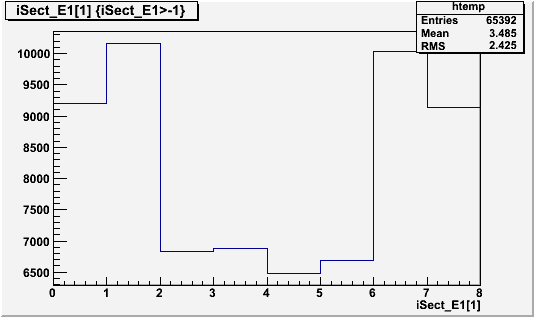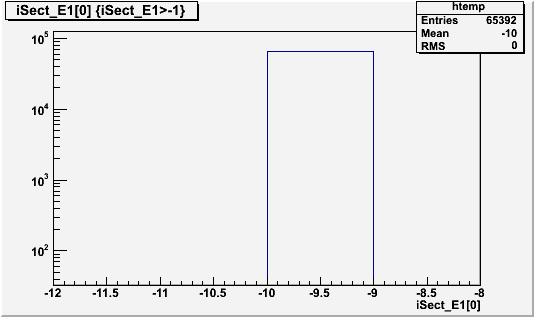# How to make a condition on all the boxes of an array?

Hello, let us suppose I have a TTree with only one leaf. This leaf contains an array called iSect_E1.

If I ask to plot something like

``t->Draw("iSect_E1","iSect_E1>-1")``

I get the following figure, i.e. without any event with value less than -1.Now, if I type

``t->Draw("iSect_E1","iSect_E1>-1")``

(0 can be replaced by 2, 3, 4, …,7)
I get the following figureHowever, if I type

``t->Draw("iSect_E1","iSect_E1>-1")``

the negative values are not plotted

Why do I get some events equal to -10 since I ask to remove the negative value with the condition “iSect_E1>-1”? Why does “iSect_E1>-1” correspond to “iSect_E1>-1” and not “iSect_E1>-1” (or anything else)?

So is there any way to write the condition so that the condition is checked on all the boxes of the array (not only the second case)? I would prefer to get an answer different of “iSect_E1>-1 && iSect_E1>-1 && …” because it could be really annoying if I have an array with dozens of boxes…